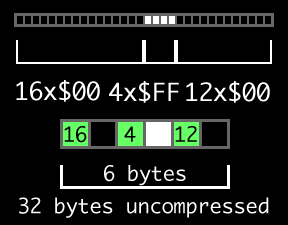# Run Length Encoding

(Redirected from RLE)
Jump to: navigation, search

Run Length Encoding (RLE) is a very simple and primitive implementation of data compression, where runs of data (sequences in which the same value occurs many times) are stored as a single set of data value and associated count, instead of the original long run of data. Obviously, RLE is very suitable for usage on data that has many of these long runs of data, such as bitmapped images with lots of empty scanlines, line drawings, mappings, etc. It is however not useful on files that do not have many continuous segments of data — the file size will actually increase significantly in this case. When decompressed, the original data will result bit per bit as it was before being compressed. This makes RLE a lossless compression. Due to it's nature, it can also be optimised to be very fast when the length count is a byte, as a Duff's Device can be used, which is an unrolled loop.

## Example SituationThe example 32 pixel scanline can be reduced to 6 with RLE.

Imagine an image that has 8 bits per pixel, used to display text. There will be many repeated pixels between rows and sometimes letters. Storing this uncompressed can be a real waste of space, and RLE is very well suited for compressing this sort of data. For the example below, imagine that W stands for white, or \$FF, and B stands for black, or \$00:

``` BBBBBBBBBBBBBBBBWWWWBBBBBBBBBBBB
```

There is many repeating instances of the black pixel colour between the four white pixels. Using the traditional RLE implementation (as well as the implementation described below) the first byte of the RLE entry will be the length counter, and the next would be the actual data. If the above data were to be compressed, the result would be as follows:

``` 16B 04W 12B
```

A real world implementation would obviously use binary to show data, but ASCII is used for clarity in this example.

## RLE Implementations

Writing an RLE implementation that handles decompression is an easy task. All that needs to be done is to loop through the file, word by word, and for every token that appears, a loop writing the specified value the number of times specified. When a byte is used for the length counter, a so-called "Duff's Device" can be used, which is an unrolled loop with 256 move instructions. Instead of a dbf-based loop, there is no more overhead from that anymore, so compression can go much faster. The number of bytes to write is first subtracted from \$FF, which is then simply shifted to the left one bit, and then the address of the start of the Duff's Device is jumped to, using the shifted byte count as an index, so that if 4 bytes need to be copied, the jump will end up 4 move instructions before the end of the device.

The total number of tokens is also often stored in the header of the RLE file as a word - this can be used to determine when to break out of the decompression loop. A sample implementation of RLE can be found below: <source lang="asm" line="GESHI_FANCY_LINE_NUMBERS">

A primitive implementation of Run Length Decompression
Arguments
|--- a5
location of compressed data
|--- a6
area to decompress to
Outputs
|--- a5
Directly after the location of compressed data
|--- a6
Directly after the area to decompress to
Destroys
d0-d3

RLEDecomp: moveq #0, d0 ; Clear d0 move.w (a5)+, d0 ; Fetch the total number of tokens moveq #0, d1 moveq #0, d2 ; Value that byte length is subtracted from moveq #1, d3 ; Bits to shift value by

@loop: move.b (a5)+, d1 ; Number of bytes to repeat the data for sub.b d1, d2 ; Subtract from the offset lsl.w d3, d2 ; Multiply by 2

move.b (a5)+, d1 ; Read out the byte to repeat.

jmp @duffsDevice(pc, d2.w) ; Jump to apt offset inside the device

@duffsDevice: rept 256 ; Assembler macro to repeat instruction contained inside it x times move.b d1, (a6)+ ; Write a byte endr

subq.w #1, d0 ; Subtract and see if we're done de-compressing. bne.s @end ; If so, branch out.

bra.w @loop ; Keep looping.

@end: rts </source>